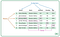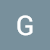# Introduction to Decision Tree Classifiers

Decision trees are a powerful tool capable of mapping non-linear relationships and complex relationships between many variables. They are also computationally inexpensive compared to other algorithms. There are many forms of decision trees, but I am going to just cover the basics of the algorithm in this post.

Decision trees work like a flow chart. The algorithm constructs a series of yes/no questions that lead to further questions until the best predictions can be produced. This is similar to the game 20 questions or the famous Akinator game. The tree starts with one question regarding the feature data. This is…

# Checking The Assumptions For Linear Regression

In this post I will talk about the assumptions for linear regression. Some must be checked before modeling begins. Others need to be checked afterward using the residuals obtained from training. It is important to check all of the assumptions for a model because failing to do so can lead to unreliable results in the real world, even if the performance metrics from evaluation on the testing set are great.

The first assumption is that the feature variables being modeled have a linear relationship with the target variable. This means that as one feature variable increases, the target variable responds…

# Introduction To The Central Limit Theorem

When conducting statistical tests, many of them require that the values of the samples are normally distributed. Often times they are, unfortunately, not normally distributed, but there is a nifty trick to fix this problem. The solution involves what is called ‘The Central Limit Theorem’.

The central limit theorem states that even if a set of data is not normally distributed, if you take many random samples with replacement and record the means of those samples, those means will be normally distributed. …

# Creating a Presentation for a Data Science Project

One of the most sought after skills in data analytics is to be able to tell a story with data. This is why you used the data visualizations, statistics, and machine learning, it was to influence business decisions. There should be plenty of domain knowledge put to use and you should convey your point in such a way that someone who has no knowledge of data analytics can understand your message. One classic way to give a presentation is with a slide show.

The first slide, after the title, should explain the problem statement. Why are you working on this…

# Why Use Cross Validation?

Cross validation is an alternative to using a validation set for machine learning models. There are several ways using cross validation instead of a stand alone validation set can improve the performance of your models. Its only drawback is that it can be computationally expensive when working with large amounts of data or with highly complex pipelines.

Cross validation involves splitting your training set into multiple equal sized pieces. Then you take all but the last one and use those for training and take the remainder and use it as a validation set. This completes one round of training and…

# K-Neighbors From Scratch

Using just python and numpy

## Why use K-Neighbors?

K-Neighbors is a good at modeling complex non-linear relationships. It is a supervised form of machine learning and is different from linear and tree based algorithms because it is distance based.

## How Does K-Neighbors Work?

K-Neighbors estimates values by examining nearby data points. A K-Neighbors model works by first saving the training data. Then when making predictions it takes each point in the testing data and finds its “neighbors” in the training data by comparing distance. The model is preset to get some number, “K”, of the neighbors. If the problem is classification it looks at the counts of…

# An Introduction to the Pandas Library

By George Bennett

Pandas is a library in python that helps with organizing and exploring data. It is built on the numpy library. Pandas stores data in neat tables called “DataFrames” that can be easily manipulated. I will be explaining how to create dataframes, how to quickly get information from dataframes with slicing, how to make quick plots, and how to export data into a .csv file.taken from https://media.geeksforgeeks.org/wp-content/uploads/finallpandas.png

To create a dataframe out of a .csv file simply call the pandas .read_csv method with the file name passed in to save it as a variable. This is the most common way…

# The Differences Between Python Lists and Numpy Arrays

By George Bennett

If your learning python you will quickly become familiar with the list datatype. Lists are an ordered collection of data, whether it be numbers, strings, collections, or any other objects. Numpy arrays are similar to lists but when dealing with numerical information they simplify mathematical processes. Numpy is a critical module to import no matter what type of work you are doing.

`# import numpy moduleimport numpy as np`

One example of numpy array magic is performing basic arithmetic. Ordinarily if you wanted to add each item in a list of numerical data with a corresponding…

# Scaling Data

By George Bennett

When using machine learning algorithms it is often a good idea to first scale the data. Scaling the data is putting all the features on a level playing field. Say you are using a distance based algorithm and you have one feature with a range in the thousands and another feature that ranges from 1 to 5. Would you want the first feature to be hundreds of times more important to the model? Scaling data puts everything in a similar range. In this post I will cover the two most common and practical ways to scale data.

# The Basics of Joining Tables

By George Bennett

Whether you are using tableau, SQL, or excel, or google sheets. It is a good Idea to know how to make joins.

Joins are used when you have two tables containing information that can be linked together. Many times in databases there will be identifier columns which act similar to an index. These are created for the sole purpose of connecting tables together if need be. Lets take an example of a business. They keep one table full of customer information like address, phone-number, and money spent. They put a customer ID in that table that is…## Datascience George

Data scientist learning at Flat Iron School